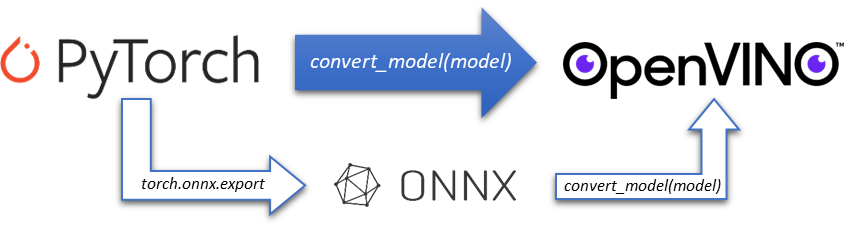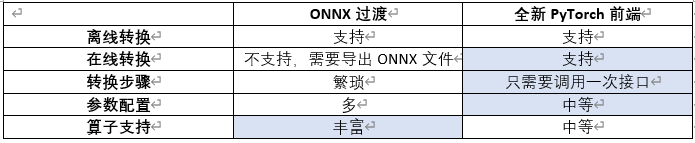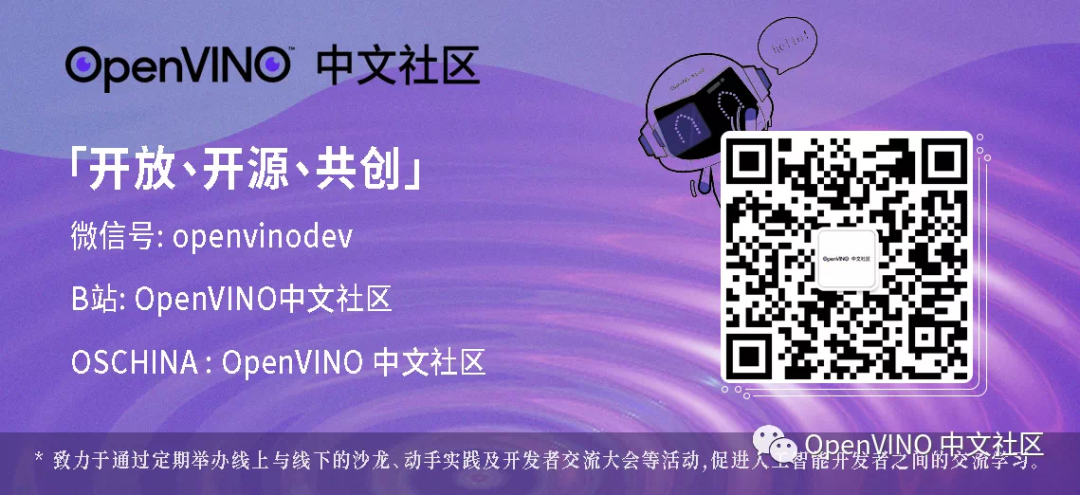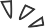# 没有“中间商赚差价”， OpenVINO™ 直接支持 PyTorch 模型对象

06/19 13:13import torchvisionimport torchfrom openvino.tools.mo import convert_modelmodel = torchvision.models.resnet50(pretrained=True)ov_model = convert_model(model)· torch.nn.Module

· torch.jit.ScriptModule

· torch.jit.ScriptFunction

import torchvisionimport torchfrom openvino.tools.mo import convert_modelmodel = torchvision.models.resnet50(pretrained=True)ov_model = convert_model(model, example_input=torch.zeros(1, 3, 100, 100))

· openvino.runtime.Tensor

· torch.Tensor

· np.ndarray

· list or tuple with tensors (openvino.runtime.Tensor / torch.Tensor / np.ndarray)

· dictionary where key is the input name, value is the tensor (openvino.runtime.Tensor / torch.Tensor / np.ndarray)

serialize(ov_model, str(ir_model_xml))

1. 获取 PyTorch 模型对象

torch_model = BertForSequenceClassification.from_pretrained(PRETRAINED_MODEL_DIR)

2. 设置模型参数并转化为 OpenVINO™ 模型对象

input_shape = PartialShape([1, -1])input_info = [("input_ids", input_shape, np.int64),("attention_mask", input_shape, np.int64),("token_type_ids", input_shape, np.int64)]default_input = torch.ones(1, MAX_SEQ_LENGTH, dtype=torch.int64)inputs = {    "input_ids": default_input,    "attention_mask": default_input,    "token_type_ids": default_input,}model = convert_model(torch_model, example_input=inputs, input=input_info)

3. 准备校验数据集，并启动量化

calibration_dataset = nncf.Dataset(data_source, transform_fn)# Quantize the model. By specifying model_type, we specify additional transformer patterns in the model.quantized_model = nncf.quantize(model, calibration_dataset,                                model_type=ModelType.TRANSFORMER)

4. 编译量化后的模型对象，并进行推理

compiled_quantized_model = core.compile_model(model=quantized_model, device_name="CPU")output_layer = compiled_quantized_model.outputsresult = compiled_quantized_model(inputs)[output_layer]result = np.argmax(result)print(f"Text 1: {sample['sentence1']}")print(f"Text 2: {sample['sentence2']}")print(f"The same meaning: {'yes' if result == 1 else 'no'}")

Text 1: Wal-Mart said it would check all of its million-plus domestic workers to ensure they were legally employed .Text 2: It has also said it would review all of its domestic employees more than 1 million to ensure they have legal status .The same meaning: yes

https://github.com/openvinotoolkit/openvino_notebooks/blob/main/notebooks/105-language-quantize-bert/105-language-quantize-bert.ipynb

--END--


你也许想了解(点击蓝字查看)⬇️

➡️ OpenVINO™ DevCon 2023重磅回归！英特尔以创新产品激发开发者无限潜能

➡️ 5周年更新 | OpenVINO™  2023.0，让AI部署和加速更容易

➡️ OpenVINO™5周年重头戏！2023.0版本持续升级AI部署和加速性能

➡️ OpenVINO™2023.0实战 | 在 LabVIEW 中部署 YOLOv8 目标检测模型

➡️ 开发者实战系列资源包来啦！

➡️

以AI作画，祝她节日快乐；简单三步，OpenVINO™ 助你轻松体验AIGC

➡️

还不知道如何用OpenVINO™作画？点击了解教程。

➡️

➡️

使用OpenVINO 在“端—边—云”快速实现高性能人工智能推理

➡️

图片提取文字很神奇？试试三步实现OCR！

➡️

【Notebook系列第六期】基于Pytorch预训练模型，实现语义分割任务

➡️

使用OpenVINO™ 预处理API进一步提升YOLOv5推理性能





扫描下方二维码立即体验
OpenVINO™ 工具套件 2023.00 评论
0 收藏
0